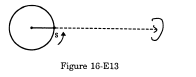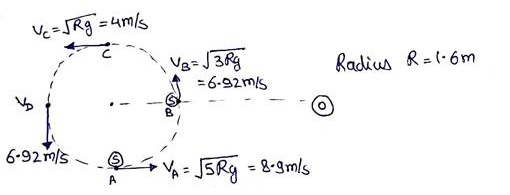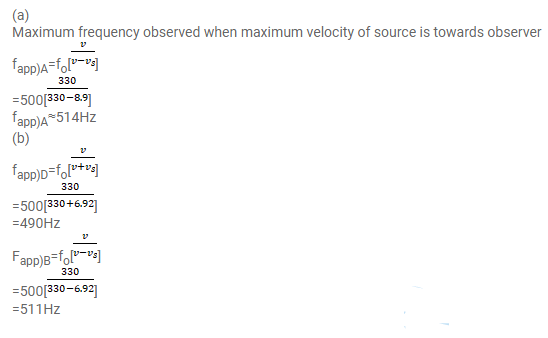# A small source of sound S of frequency 500 HzQuestion:

A small source of sound $S$ of frequency $500 \mathrm{~Hz}$ is attached to the end of a light string and is whirled in a vertical circle of radius $1.6 \mathrm{~m}$. The string just remains tight when the source is at the highest point. (a) An observer is located in the same vertical plane at a large distance and at the same height as the centre of the circle. The speed of sound in air $=330 \mathrm{~m} \mathrm{~s}^{-1}$ and $g=10 \mathrm{~m} \mathrm{~s}^{-2}$. Find the maximum frequency heard by the observer. (b) An observer is situated at a large distance vertically above the centre of the circle. Find the frequency heard by the observer corresponding to the sound emitted by the source when it is at the same height as the centre.Solution: